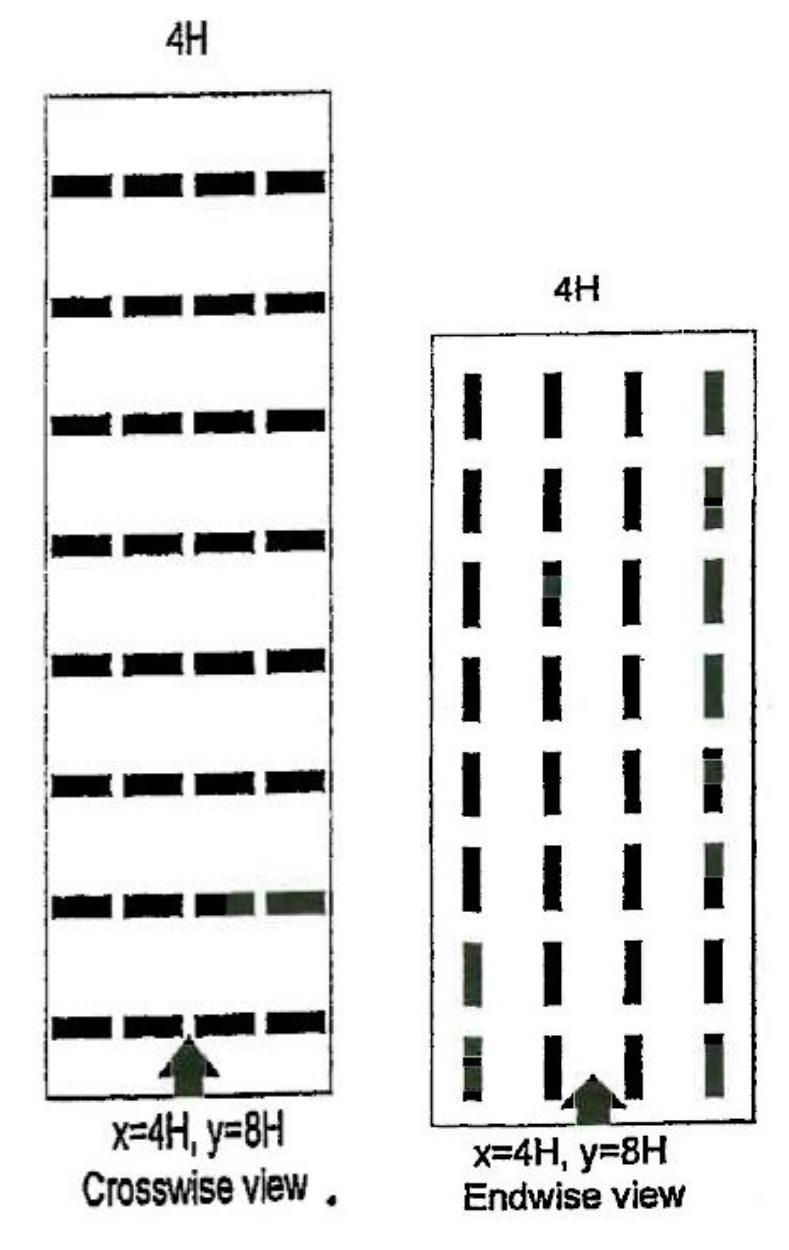What is the UGR method in DIALux?

The UGR value is a measure of glare. The greater the value, the more glare the light source causes. The UGR observer is at a height of 1.2 metres as standard. Its viewing direction is in DIALux (cos(α), sin(α), 0), where α is the angle to the x-axis.

UGR values are calculated in DIALux using the following formula (see CIE 117).Bezeichnungen:

 L Luminance [cd / m2] r Distance of the luminaire to the observer [m] I Luminous intensity [cd] Ap projected area of the luminous parts [m2] ω Solid angle of the luminous parts [sr] = Ap / r2 p Position index of the LEO Eind indirect illuminance at the observer's eye [lux] Lb Background luminance [cd / m2] = Eind / π n Number of LEOs in the scene

The position index is determined as follows:

First, the R, T and H coordinates of the luminaires are determined with respect to the observer. Then the position index is calculated from the table by means of interpolation. Luminaires with a position index outside the validity range of the table are not considered in the UGR calculation. This is the case, for example, in H/R > 2.

#### What are UGR tables?

With UGR tables, rooms of the dimensions (2H, 2H),...(12H, 8H) are equipped with luminaire fields. H is always 2m for UGR tables. The distance between the luminaires is determined by the "Space To Height Ratio" (SHR). Permissible values are 0.25 and 1, so with SHR = 1 the luminaire spacing is 2m, with SHR = 0.25 it is 0.5m. The observer is always in the middle of the wall at a height of 1.2m (see sketches). Furthermore, there is the arrangement viewing direction transverse to the lamp axis and viewing direction longitudinal to the lamp axis.Please have also a look at the following article on our DIAL website: No glare from artificial light. What is behind the UGR method?

and the tutorial about UGR calculation on our YouTube channel: UGR calculation in evo